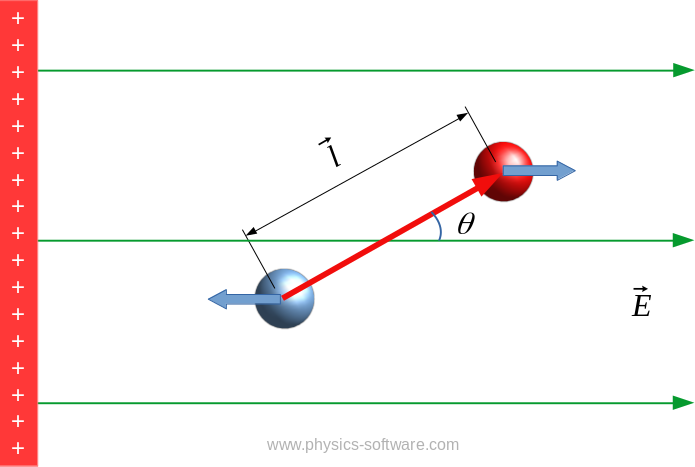Dipole in uniform electric fieldDipole moment is:  $\vec{p} = q \vec{l}$.
Here $q$ is charge,  $\vec{l}$ is distance between charges.

Torque on dipole: $$\vec{M}=\vec{p} \times \vec{E}$$